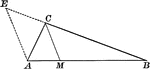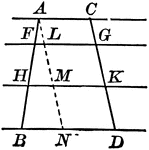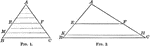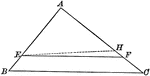### Angle Bisector of Triangle Dividing Opposite Side Into Proportionate Segments

Illustration to show that an angle bisector of a triangle divides the opposite side into segments which…### Exterior Angle Bisector of Triangle Dividing Opposite Side Into Proportionate Segments

Illustration to show that a bisector of a an exterior angle of a triangle divides the opposite side…### Parallel Lines Cut by Parallels, Corresponding Intercepts Proportional

Illustration to show that if parallel lines are cut by any number of parallels, the corresponding intercepts…### Triangle Divided by Parallel Lines to Form Proportionate Triangles

Illustration to show that if a line is drawn through two sides of a triangle parallel to the third side,…### Triangle Divided by Parallel Lines to Form Proportionate Triangles

Illustration to show that if a line divides two sides of a triangle proportionally, it is parallel to…